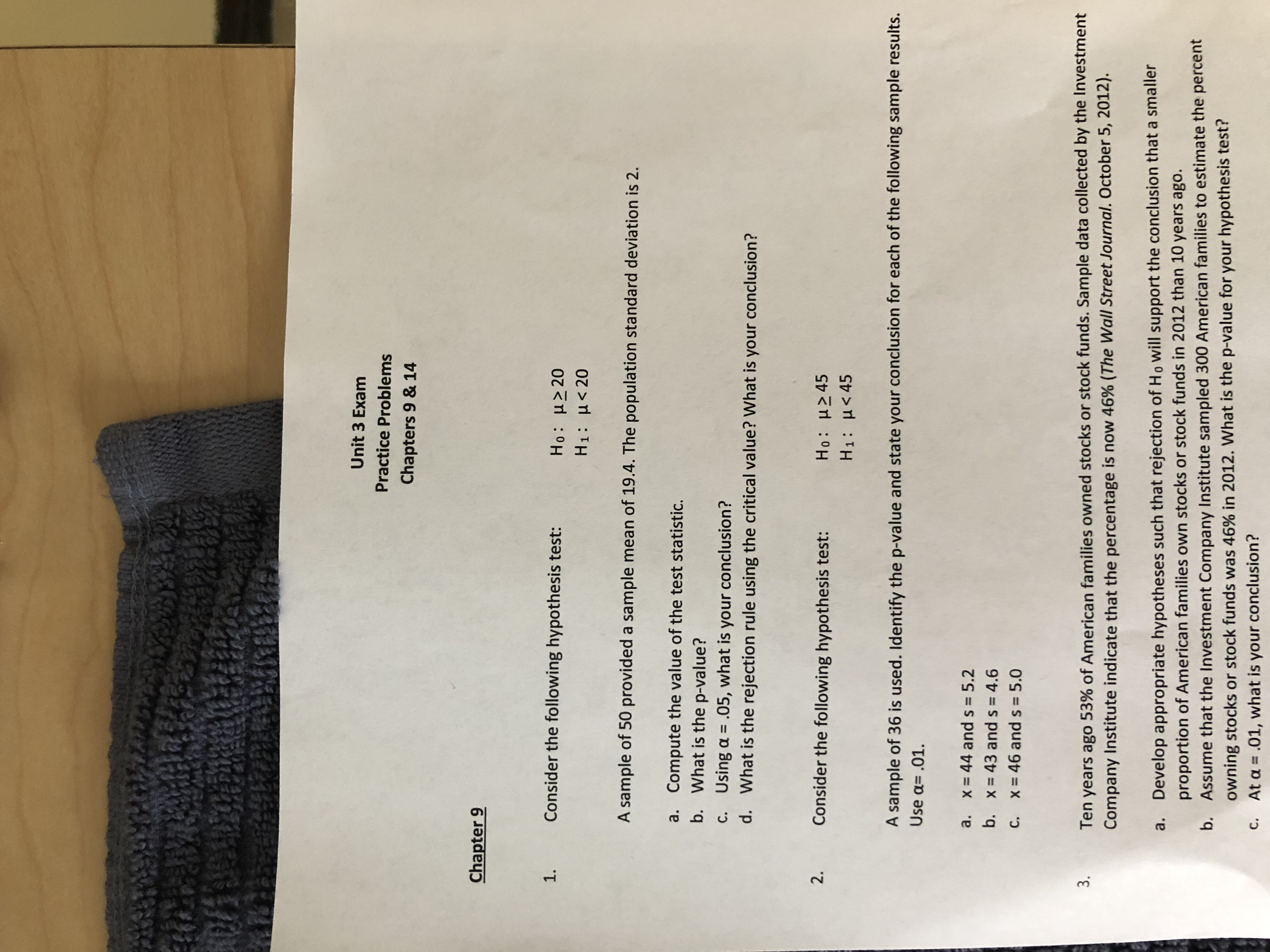# VECUnit 3 ExamPractice ProblemsChapters 9 & 14Chapter 91Consider the following hypothesis test:Ho: μ>20H1: H

Question
14 views

Number 1 Please, Explain shortcut with TI84 if applicablehelp_outlineImage TranscriptioncloseVEC Unit 3 Exam Practice Problems Chapters 9 & 14 Chapter 9 1 Consider the following hypothesis test: Ho: μ>20 H1: H<20 A sample of 50 provided a sample mean of 19.4. The population standard deviation is 2 Compute the value of the test statistic. What is the p-value? a. b. Using a .05, what is your conclusion? What is the rejection rule using the critical value? What is your conclusion? C. d. 2. Consider the following hypothesis test: Ho: H245 H1: H 45 A sample of 36 is used. Identify the p-value and state your conclusion for each of the following sample results. Use a .01. a. x 44 and s = 5.2 b. x 43 and s = 4.6 46 and s = 5.0 c. x Ten years ago 53% of American families owned stocks or stock funds. Sample data collected by the Investment Company Institute indicate that the percentage is now 46% (The Wall Street Journal. October 5, 2012) 3. Develop appropriate hypotheses such that rejection of Ho will support the conclusion that a smaller proportion of American families own stocks or stock funds in 2012 than 10 years ago. a. Assume that the Investment Company Institute sampled 300 American families to estimate the percent owning stocks or stock funds was 46 % in 2012. What is the p-value for your hypothesis test? At a .01, what is your conclusion? b. C. fullscreen
check_circle

Step 1

a. Computation of test statistic value:

Sample size is greater than 30 and the population standard deviation is given. Thus, in order to test H0: µ ≥ 20 vs H1: µ < 20, the appropriate test is one sample z test.

The formula for one sample z test is given below.

Step 2

Calculation:

Here, x = 19.4, µ = 20, σ = 2, and n is 50. By substituting these values in z formula, the test statistic value is |z| = 2.1213.

Step 3

b. Computation of p-value:

The EXCEL formula to find the p-value for one-tailed z-test is “=1-NORM.S.DIST(...

### Want to see the full answer?

See Solution

#### Want to see this answer and more?

Solutions are written by subject experts who are available 24/7. Questions are typically answered within 1 hour.*

See Solution
*Response times may vary by subject and question.
Tagged in
MathStatistics

### Hypothesis Testing